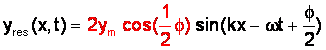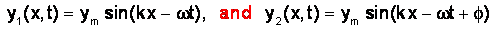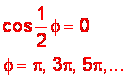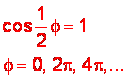# 1-9 Interference of wavesTwo sinusoidal waves y1(x,t) and y2(x,t)

### Constructive and Destructive interference

moving in the same medium interfere to produce the resultant wave yres(x,t)We say that the two waves interfere destructively if the resultant wave has an amplitude of zero, which is the minimum possible amplitude the resultant wave can have. Thus destructive interference occurs when

Destructive interference
occurs when the phase difference between wave 1 and wave 2 is an odd integer multiple of p,or equivalently, the path difference between them is an odd integer multiple of l/2.We say that the two waves interfere constructively if the resultant wave has an amplitude of 2ym, which is the maximum possible amplitude the resultant wave can have. Thus constructive interference occurs when

Constructive interference occurs when the phase difference between wave 1 and wave 2 is an integer multiple of 2p,or equivalently, the path difference between them is an integer multiple of l.